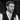# A More Idiomatic Two-Sum Solution in JavaScript

Nick Scialli August 26, 2019🚀 3 minute read

If you're enjoying this blog, please consider one or both of the following:

# Introduction

A couple weeks ago, I explored the two-sum coding challenge question and discussed both inefficient and efficient ways to solve it. Today, I improve on the efficient solution by providing a solution that’s a bit more idiomatic to JavaScript.

# The Two-Sum Question

First, let’s understand the two-sum question. It’s usually posed as some form of the following:

You are asked to create a function that takes two parameters. The first parameter, `nums`, is an array of numbers. The second parameter, `total` is a single number. The output of the function should be a two-element array that represents a pair of numbers in `nums` that add up to `total`.

``````/**
* @param {number[]} nums
* @param {number} total
* @return {number[]}
*/
const twoSum = (arr, total) => {
// Solution here
};``````

Typically, we’re given a couple examples of valid input/output combinations:

``````input: nums = [1, 2, 3], total = 4
output: [1, 3]

input: nums = [3, 9, 12, 20], total = 21
output: [9, 12]``````

# The Previous Solution

In my previous blog post, I arrived at the following solution. It only requires iterating through the array once, adding elements to an object as it goes. It then searches that object for a “complement” value to go with the current array element. Due to hash table efficieny of objects, the solution is O(n) time complexity.

``````const twoSum = (nums, total) => {
const previousValues = {};
for (let i = 0; i < nums.length; i++) {
const complement = total - nums[i];
if (previousValues[complement]) {
return [complement, nums[i]];
}
previousValues[nums[i]] = true;
}
};``````

# A More Idiomatic Way

JavaScript actually has a specific object to that would help with this problem: the `Set` object. `Set` is “more idiomatic” because it’s a mechanism to store unique values (or object references) without having to do the weird `previousValues[nums[i]] = true;` workaround I did above.

If we change our implementation to use `Set`, it might look as follows:

``````const twoSum = (nums, total) => {
const previousValues = new Set();
for (let i = 0; i < nums.length; i++) {
const complement = total - nums[i];
if (previousValues.has(complement)) {
return [complement, nums[i]];
}
}
};``````

According to the EcmaScript 2015 spec, “Set objects must be implemented using either hash tables or other mechanisms that, on average, provide access times that are sublinear on the number of elements in the collection.” So, we’re not necessarily sure `Set` will be implemented using has tables, but we can be confident of its efficiency.

# Conclusion

Both the object cache and `Set` methods will solve the two-sum problem efficiently, but Sets seem to be the more idiomatic way to approach the problem in JavaScript!Nick Scialli is a software engineer at the U.S. Digital Service.

### Subscribe to the mailing list!

If you like what I post here, please sign up to get updates and code insights in your inbox. I won't spam you and you can unsubscribe any time!